# 4.6. Global Ocean Simulation in Pressure Coordinates¶

This example experiment demonstrates using MITgcm to simulate the planetary ocean circulation in pressure coordinates, that is, without making the Boussinesq approximations. The simulation is configured as a near copy of tutorial_global_oce_latlon (Section 4.5). with realistic geography and bathymetry on a $$4^{\circ} \times 4^{\circ}$$ spherical polar grid. Fifteen levels are used in the vertical, ranging in thickness from 50.4089 dbar $$\approx$$ 50 m at the surface to 710.33 dbar $$\approx$$ 690 m at depth, giving a maximum model depth of 5302.3122 dbar $$\approx$$ 5200 m. At this resolution, the configuration can be integrated forward for thousands of years on a single processor desktop computer.

## 4.6.1. Overview¶

The model is forced with climatological wind stress data from Trenberth (1990) [TOL90] and surface flux data from Jiang et al. (1999) [JSMR99]. Climatological data (Levitus and Boyer 1994a,b [LB94a][LB94b]) is used to initialize the model hydrography. Levitus and Boyer seasonal climatology data is also used throughout the calculation to provide additional air-sea fluxes. These fluxes are combined with the Jiang et al. climatological estimates of surface heat flux, resulting in a mixed boundary condition of the style described in Haney (1971) [Han71]. Altogether, this yields the following forcing applied in the model surface layer.

(4.42)${\cal F}_{u} = g\frac{\tau_{x}}{\Delta p_{s}}$
(4.43)${\cal F}_{v} = g\frac{\tau_{y}}{\Delta p_{s}}$
(4.44)${\cal F}_{\theta} = - g\lambda_{\theta} ( \theta - \theta^{\ast} ) - \frac{1}{C_{p} \Delta p_{s}}{\cal Q}$
(4.45)${\cal F}_{s} = + g\rho_{FW}\frac{S}{\rho\Delta p_{s}}({\cal E} - {\cal P} - {\cal R})$

where $${\cal F}_{u}$$, $${\cal F}_{v}$$, $${\cal F}_{\theta}$$, $${\cal F}_{s}$$ are the forcing terms in the zonal and meridional momentum and in the potential temperature and salinity equations respectively. The term $$\Delta p_{s}$$ represents the top ocean layer thickness in Pa. It is used in conjunction with a reference density, $$\rho_{FW}$$ (here set to 999.8 kg m-3), the surface salinity, $$S$$, and a specific heat capacity, $$C_{p}$$ (here set to 4000 J kg-1 K-1), to convert input dataset values into time tendencies of potential temperature (with units of oC s-1), salinity (with units ppt s-1) and velocity (with units m s-2). The externally supplied forcing fields used in this experiment are $$\tau_{x}$$, $$\tau_{y}$$, $$\theta^{\ast}$$, $$\cal{Q}$$ and $$\cal{E}-\cal{P}-\cal{R}$$. The wind stress fields ($$\tau_x$$, $$\tau_y$$) have units of N m-2. The temperature forcing fields ($$\theta^{\ast}$$ and $$Q$$) have units of oC and W m-2 respectively. The salinity forcing fields ($$\cal{E}-\cal{P}-\cal{R}$$) has units of m s-1 respectively. The source files and procedures for ingesting these data into the simulation are described in the experiment configuration discussion in section Section 4.5.3.

## 4.6.2. Discrete Numerical Configuration¶

Due to the pressure coordinate, the model can only be hydrostatic (de Szoeke and Samelson 2002 [dSS02]). The domain is discretized with a uniform grid spacing in latitude and longitude on the sphere $$\Delta \phi=\Delta \lambda=4^{\circ}$$, so that there are 90 grid cells in the zonal and 40 in the meridional direction. The internal model coordinate variables $$x$$ and $$y$$ are initialized according to

\begin{split}\begin{aligned} x=r\cos(\phi),~\Delta x & = &r\cos(\Delta \phi) \\ y=r\lambda,~\Delta y &= &r\Delta \lambda \end{aligned}\end{split}

Arctic polar regions are not included in this experiment. Meridionally the model extends from 80oS to 80oN. Vertically the model is configured with fifteen layers with the following thicknesses

$$\Delta p_{1}$$ = 7103300.720021 Pa
$$\Delta p_{2}$$ = 6570548.440790 Pa
$$\Delta p_{3}$$ = 6041670.010249 Pa
$$\Delta p_{4}$$ = 5516436.666057 Pa
$$\Delta p_{5}$$ = 4994602.034410 Pa
$$\Delta p_{6}$$ = 4475903.435290 Pa
$$\Delta p_{7}$$ = 3960063.245801 Pa
$$\Delta p_{8}$$ = 3446790.312651 Pa
$$\Delta p_{9}$$ = 2935781.405664 Pa
$$\Delta p_{10}$$ = 2426722.705046 Pa
$$\Delta p_{11}$$ = 1919291.315988 Pa
$$\Delta p_{12}$$ = 1413156.804970 Pa
$$\Delta p_{13}$$ = 1008846.750166 Pa
$$\Delta p_{14}$$ = 705919.025481 Pa
$$\Delta p_{15}$$ = 504089.693499 Pa

(here the numeric subscript indicates the model level index number, $${\tt k}$$; note that the surface layer has the highest index number 15) to give a total depth, $$H$$, of -5200 m. In pressure, this is $$p_{b}^{0}$$ = 53023122.566084 Pa. The implicit free surface form of the pressure equation described in Marshall et al. (1997) [MHPA97] with the nonlinear extension by Campin et al. (2004) [CAHM04] is employed. A Laplacian operator, $$\nabla^2$$, provides viscous dissipation. Thermal and haline diffusion is also represented by a Laplacian operator.

Wind-stress forcing is added to the momentum equations in (4.46) for both the zonal flow, $$u$$ and the meridional flow $$v$$, according to equations (4.42) and (4.43). Thermodynamic forcing inputs are added to the equations in (4.47) for potential temperature, $$\theta$$, and salinity, $$S$$, according to equations (4.44) and (4.45). This produces a set of equations solved in this configuration as follows:

(4.46)$\begin{split}\frac{Du}{Dt} - fv + \frac{1}{\rho}\frac{\partial \Phi^{'}}{\partial x} - \nabla_{h}\cdot A_{h}\nabla_{h}u - (g\rho_0)^2\frac{\partial}{\partial p}A_{r}\frac{\partial u}{\partial p} &= \begin{cases} {\cal F}_u & \text{(surface)} \\ 0 & \text{(interior)} \end{cases} \\ \frac{Dv}{Dt} + fu + \frac{1}{\rho}\frac{\partial \Phi^{'}}{\partial y} - \nabla_{h}\cdot A_{h}\nabla_{h}v - (g\rho_0)^2\frac{\partial}{\partial p}A_{r}\frac{\partial v}{\partial p} &= \begin{cases} {\cal F}_v & \text{(surface)} \\ 0 & \text{(interior)} \end{cases}\end{split}$
$\frac{\partial p_{b}}{\partial t} + \nabla_{h}\cdot \vec{u} = 0$
(4.47)$\begin{split}\frac{D\theta}{Dt} - \nabla_{h}\cdot K_{h}\nabla_{h}\theta - (g\rho_0)^2\frac{\partial}{\partial p}\Gamma(K_{r})\frac{\partial\theta}{\partial p} &= \begin{cases} {\cal F}_\theta & \text{(surface)} \\ 0 & \text{(interior)} \end{cases} \\ \frac{D s}{Dt} - \nabla_{h}\cdot K_{h}\nabla_{h}s - (g\rho_0)^2\frac{\partial}{\partial p}\Gamma(K_{r})\frac{\partial S}{\partial p} &= \begin{cases} {\cal F}_s & \text{(surface)} \\ 0 & \text{(interior)} \end{cases}\end{split}$
$\Phi_{-H}'^{(0)} + \alpha_{0}p_{b}+ \int^{p}_{0}\alpha' dp = \Phi'$

where $$u=\frac{Dx}{Dt}=r \cos(\phi)\frac{D \lambda}{Dt}$$ and $$v=\frac{Dy}{Dt}=r \frac{D \phi}{Dt}$$ are the zonal and meridional components of the flow vector, $$\vec{u}$$, on the sphere. As described in Section 2, the time evolution of potential temperature $$\theta$$ equation is solved prognostically. The full geopotential height $$\Phi$$ is diagnosed by summing the geopotential height anomalies $$\Phi'$$ due to bottom pressure $$p_{b}$$ and density variations. The integration of the hydrostatic equation is started at the bottom of the domain. The condition of $$p=0$$ at the sea surface requires a time-independent integration constant for the height anomaly due to density variations $$\Phi_{-H}'^{(0)}$$, which is provided as an input field.

## 4.6.3. Experiment Configuration¶

The experiment files

contain the code customizations and parameter settings for these experiments. Below we describe the customizations to these files associated with this experiment.

### 4.6.3.1. Driving Datasets¶

Figure 4.32-Figure 4.37 show the relaxation temperature ($$\theta^{\ast}$$) and salinity ($$S^{\ast}$$) fields, the wind stress components ($$\tau_x$$ and $$\tau_y$$), the heat flux ($$Q$$) and the net fresh water flux ($${\cal E} - {\cal P} - {\cal R}$$) used in equations (4.42) - (4.45). The figures also indicate the lateral extent and coastline used in the experiment. Figure 4.38 shows the depth contours of the model domain.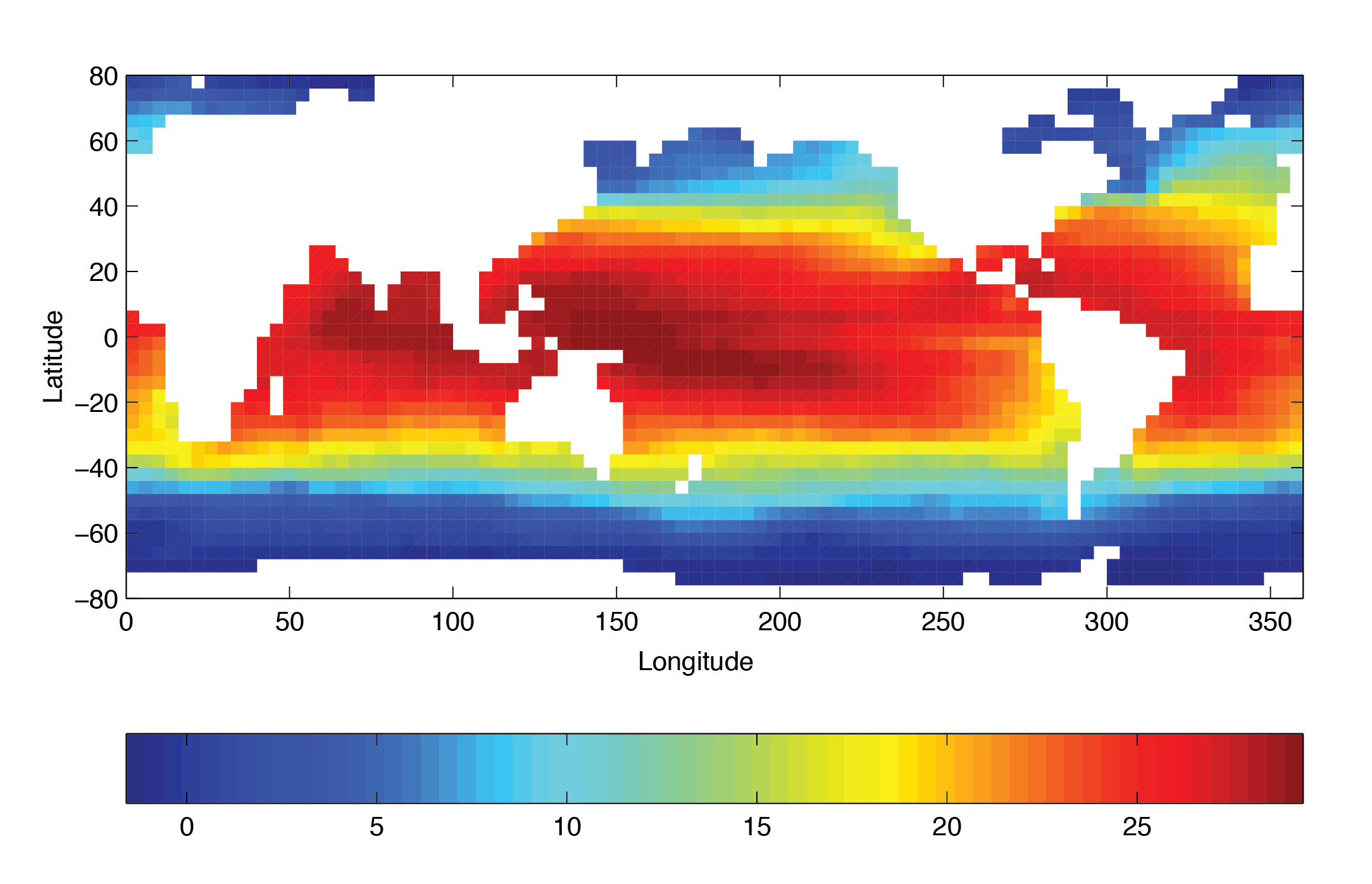Figure 4.32 Annual mean of relaxation temperature (oC)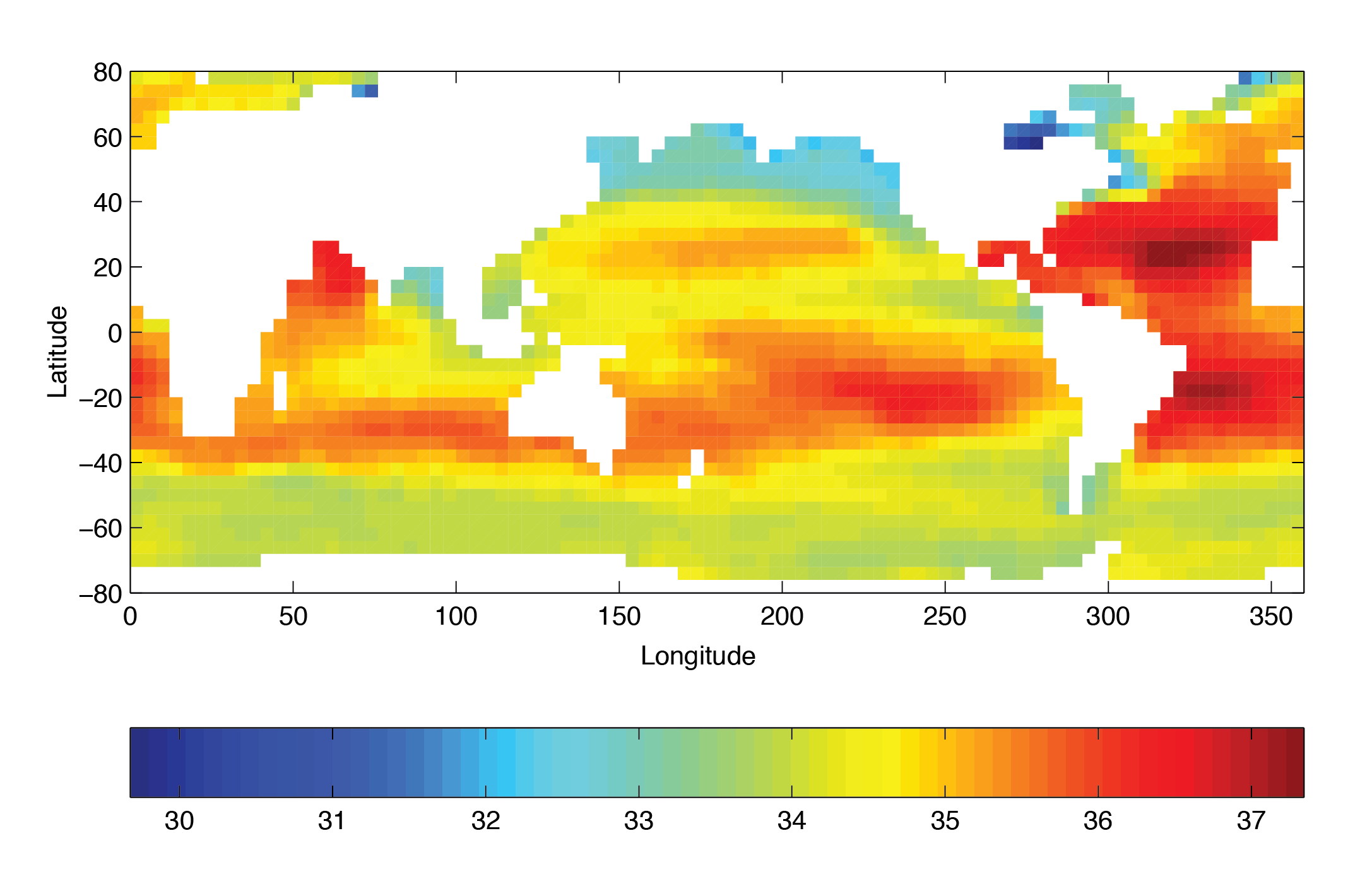Figure 4.33 Annual mean of relaxation salinity (psu)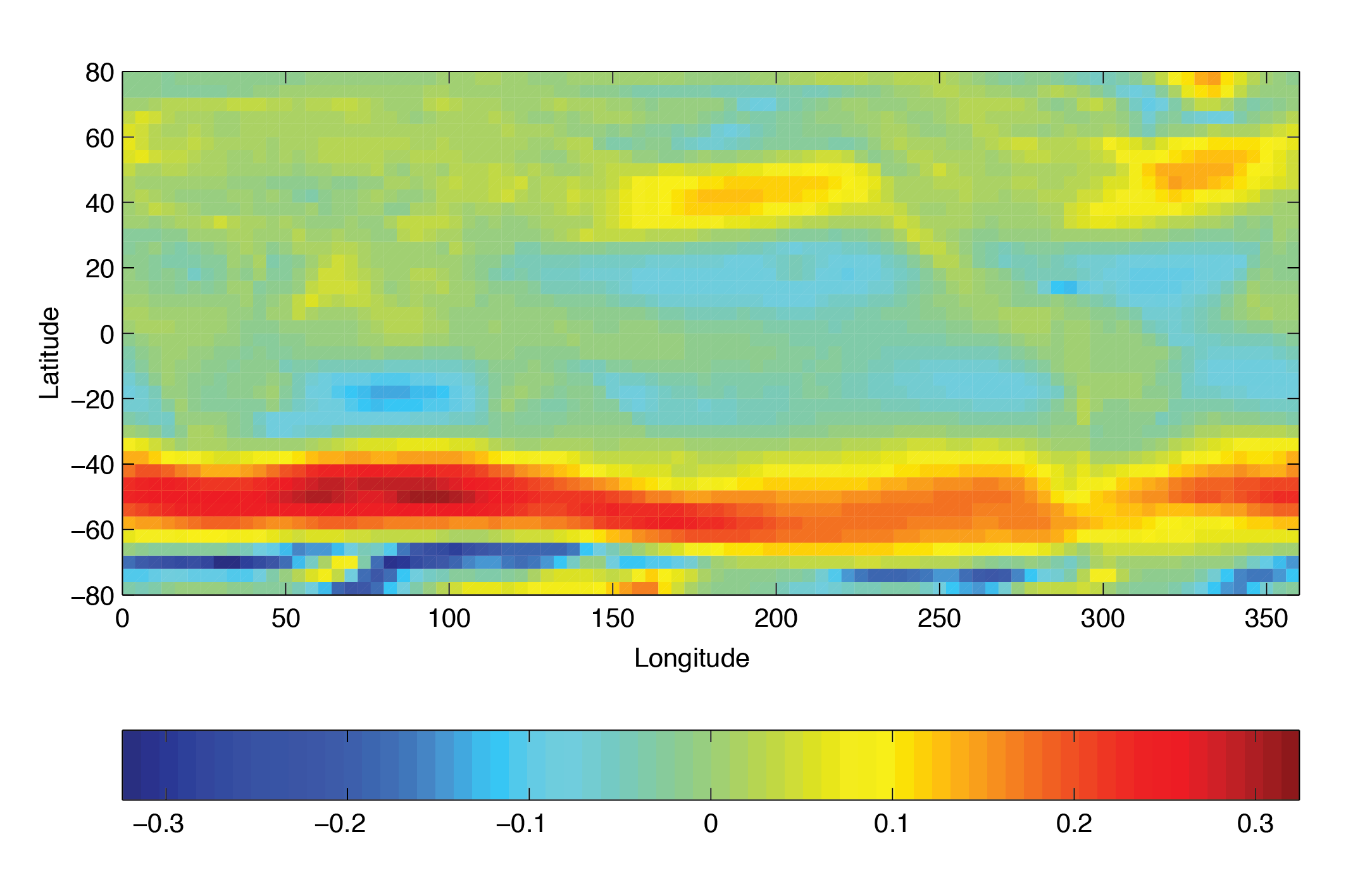Figure 4.34 Annual mean of zonal wind stress component (N m-2)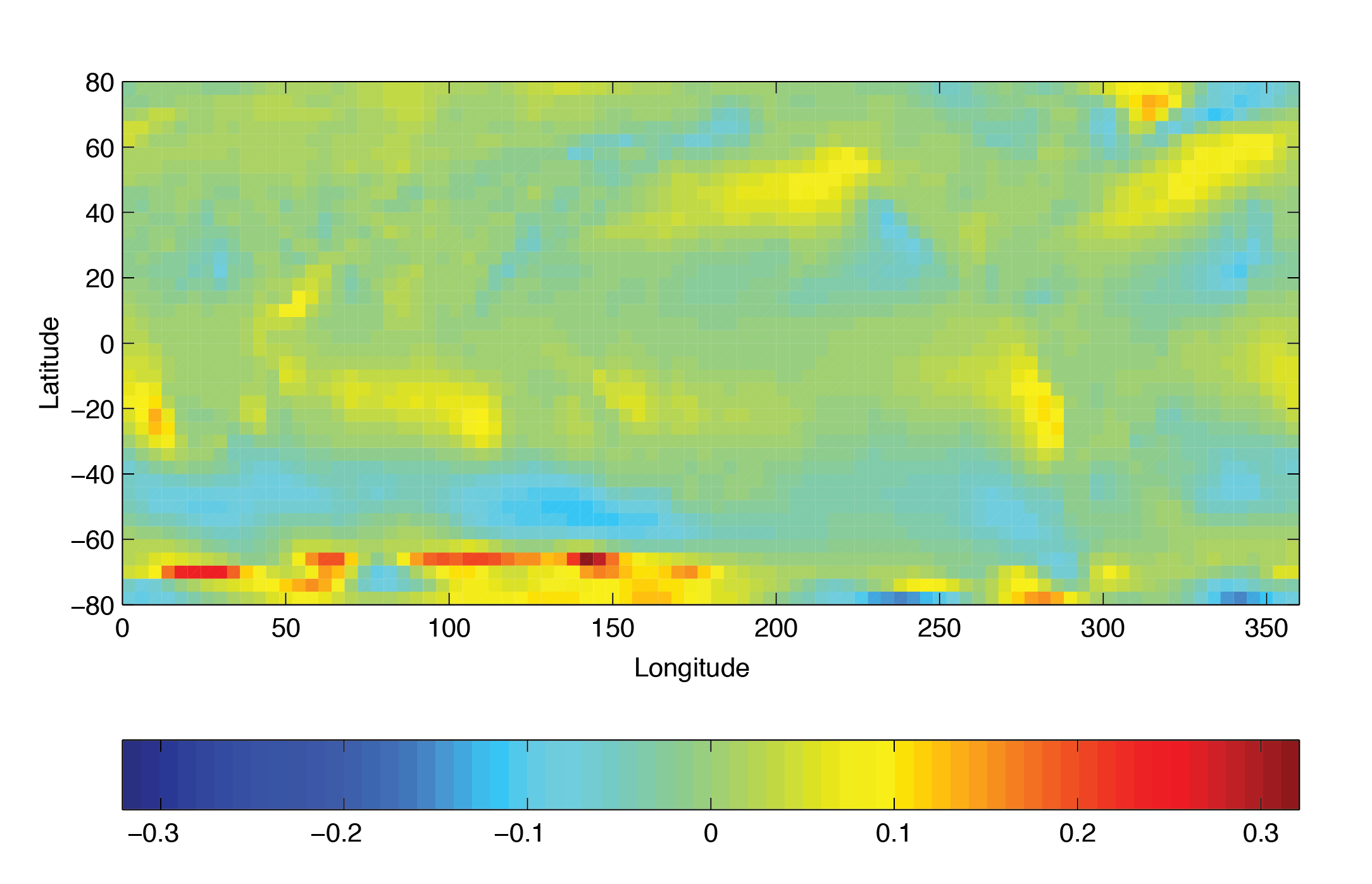Figure 4.35 Annual mean of meridional wind stress component (N m-2)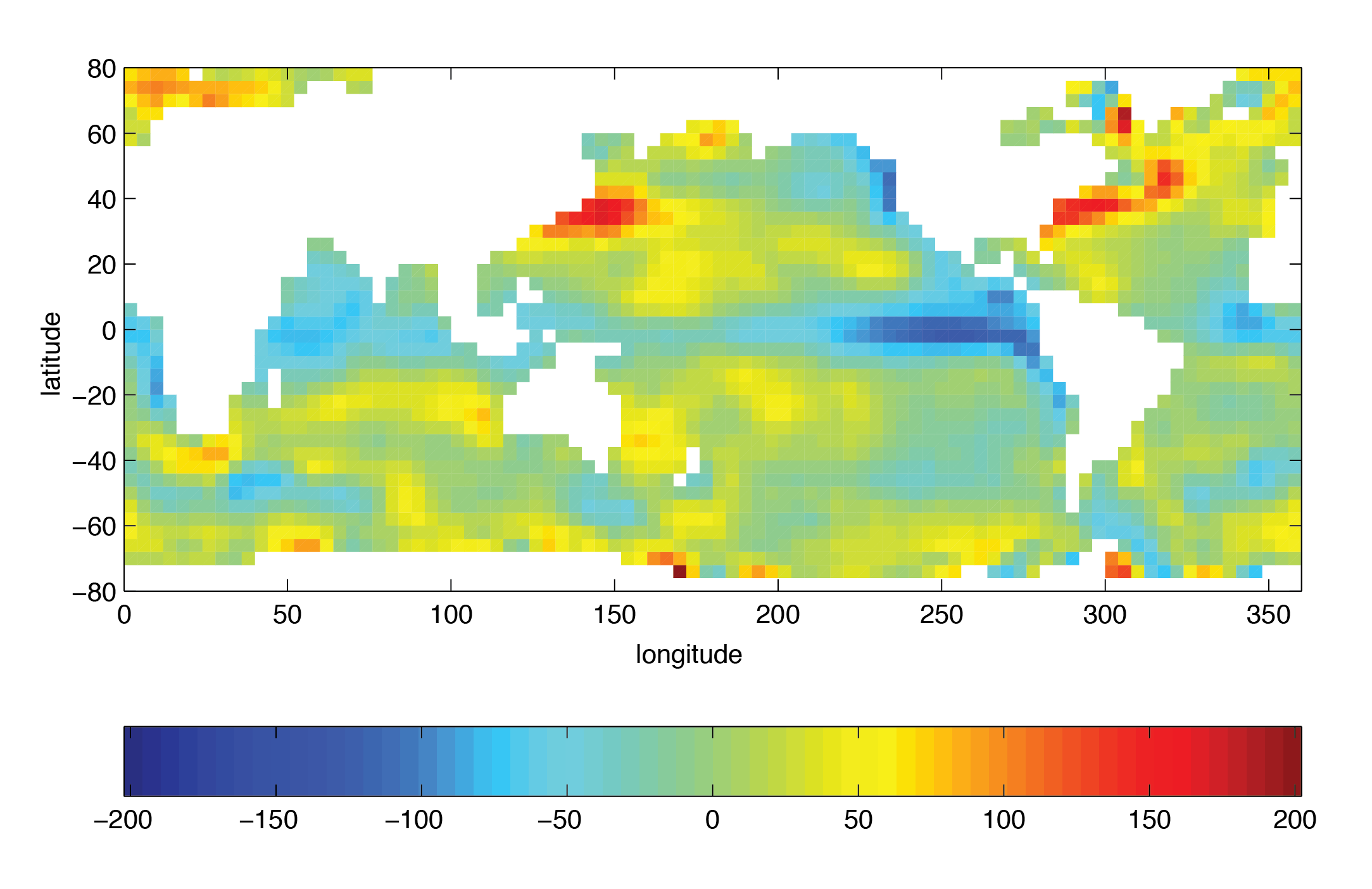Figure 4.36 Annual mean heat flux (W m-2)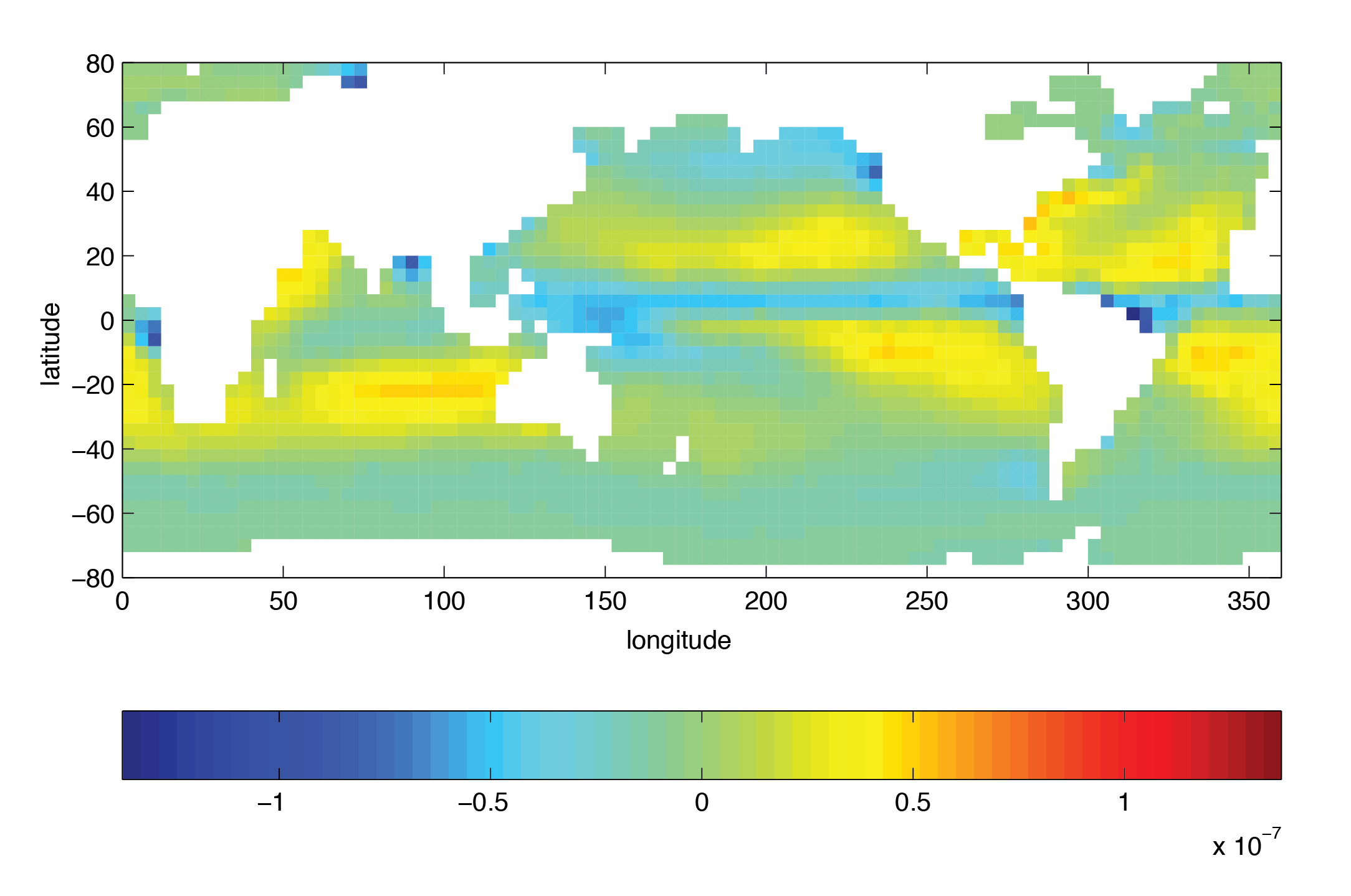Figure 4.37 Annual mean freshwater flux (Evaporation-Precipitation) (m s-1)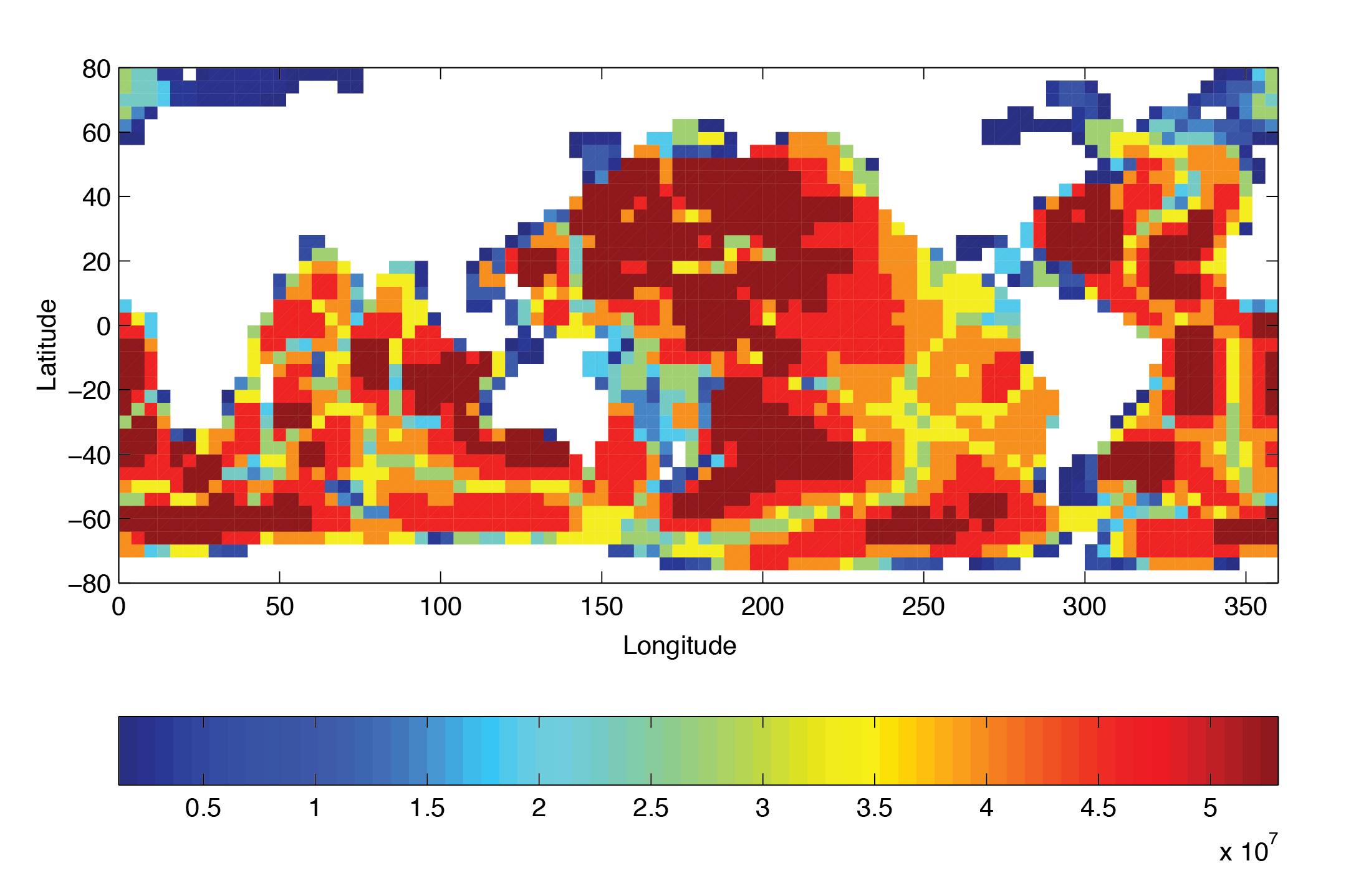Figure 4.38 Model bathymetry in pressure units (Pa)

### 4.6.3.2. File input/data¶

Listing 4.25 verification/tutorial_global_oce_oce_in_p/input/data
  1 2 3 4 5 6 7 8 9 10 11 12 13 14 15 16 17 18 19 20 21 22 23 24 25 26 27 28 29 30 31 32 33 34 35 36 37 38 39 40 41 42 43 44 45 46 47 48 49 50 51 52 53 54 55 56 57 58 59 60 61 62 63 64 65 66 67 68 69 70 71 72 73 74 75 76 77 78 79 80 81 82 83 84 85 86 87 88 89 90 91 92 93 94 95 96 97 # ==================== # | Model parameters | # ==================== # # Continuous equation parameters &PARM01 tRef = 15*20., sRef = 15*35., viscAh =3.E5, no_slip_sides=.TRUE., viscAr =1.721611620915750e5, #viscAz =1.67E-3, no_slip_bottom=.FALSE., diffKhT=1.E3, diffKrT=5.154525811125000e3, #diffKzT=0.5E-4, diffKhS=1.E3, diffKrS=5.154525811125000e3, #diffKzS=0.5E-4, cosPower=0.5, implicitDiffusion=.TRUE., ivdc_kappa=1.030905162225000e9, #ivdc_kappa=10.0, gravity=9.81, rhoConst=1035., rhoConstFresh=1000., buoyancyRelation='OCEANICP', integr_GeoPot=1, eosType='JMD95P', useNHMTerms=.TRUE., implicitFreeSurface=.TRUE., exactConserv=.TRUE., nonlinFreeSurf=4, hFacInf=0.2, hFacSup=2.0, #- to check that it has no impact: doResetHFactors=.TRUE., #useRealFreshWaterFlux=.TRUE., readBinaryPrec=64, writeBinaryPrec=64, & # Elliptic solver parameters &PARM02 cg2dMaxIters=200, cg2dTargetResidual=1.E-9, & # Time stepping parameters &PARM03 startTime = 0., endTime = 3456000., # after 100 years of intergration, one gets a reasonable flow field #endTime = 3110400000., deltaTMom = 1200.0, deltaTtracer = 172800.0, deltaTFreeSurf = 172800.0, deltaTClock = 172800.0, abEps = 0.1, pChkptFreq = 3110400000., dumpFreq = 3110400000., taveFreq = 3110400000., monitorFreq = 1., periodicExternalForcing=.TRUE., externForcingPeriod=2592000., externForcingCycle=31104000., tauThetaClimRelax=5184000.0, & # Gridding parameters &PARM04 usingSphericalPolarGrid=.TRUE., dxSpacing=4., dySpacing=4., #Ro_SeaLevel=53023122.566084, top_Pres=1.E-6, delR=7103300.720021, 6570548.440790, 6041670.010249, 5516436.666057, 4994602.034410, 4475903.435290, 3960063.245801, 3446790.312651, 2935781.405664, 2426722.705046, 1919291.315988, 1413156.804970, 1008846.750166, 705919.025481, 504089.693499, ygOrigin=-80., & # Input datasets &PARM05 bathyFile ='topog.bin', pLoadFile ='deltageopotjmd95.bin', hydrogThetaFile='lev_t.bin', hydrogSaltFile ='lev_s.bin', zonalWindFile ='trenberth_taux.bin', meridWindFile ='trenberth_tauy.bin', thetaClimFile ='lev_sst.bin', #saltClimFile ='lev_sss.bin', surfQnetFile ='shi_qnet.bin', EmPmRFile ='shi_empmr.bin', & 

This file specifies the main parameters for the experiment. The parameters that are significant for this configuration are

• Line 9–10,

viscAh=3.E5,
no_slip_sides=.TRUE.


these lines set the horizontal Laplacian frictional dissipation coefficient to $$3 \times 10^{5}$$ m2 s-1 and specify a no-slip boundary condition for this operator, i.e., $$u=0$$ along boundaries in $$y$$ and $$v=0$$ along boundaries in $$x$$.

• Lines 11-13,

 viscAr =1.721611620915750e5,
#viscAz =1.67E-3,
no_slip_bottom=.FALSE.,


These lines set the vertical Laplacian frictional dissipation coefficient to $$1.721611620915750 \times 10^{5}$$ Pa2 s-1, which corresponds to $$1.67\times10^{-3}$$ m2 s-1 in the commented line, and specify a free slip boundary condition for this operator, i.e., $$\frac{\partial u}{\partial p}=\frac{\partial v}{\partial p}=0$$ at $$p=p_{b}^{0}$$, where $$p_{b}^{0}$$ is the local bottom pressure of the domain at rest. Note that the factor $$(g\rho)^2$$ needs to be included in this value.

• Line 14,

diffKhT=1.E3,


this line sets the horizontal diffusion coefficient for temperature to 1000 m2 s-1. The boundary condition on this operator is $$\frac{\partial}{\partial x}=\frac{\partial}{\partial y}=0$$ on all boundaries.

• Line 15–16,

 diffKrT=5.154525811125000e3,
#diffKzT=0.5E-4,


this line sets the vertical diffusion coefficient for temperature to $$5.154525811125 \times 10^{3}$$ Pa2 s-1, which corresponds to $$5\times10^{-4}$$ m2 s-1 in the commented line. Note that the factor $$(g\rho)^2$$ needs to be included in this value. The boundary condition on this operator is $$\frac{\partial}{\partial p}=0$$ at both the upper and lower boundaries.

• Line 17–19,

 diffKhS=1.E3,
diffKrS=5.154525811125000e3,
#diffKzS=0.5E-4,


These lines set the diffusion coefficients for salinity to the same value as for temperature.

• Line 21–23,

 implicitDiffusion=.TRUE.,
ivdc_kappa=1.030905162225000E9,
#ivdc_kappa=10.0,


Select implicit diffusion as a convection scheme and set coefficient for implicit vertical diffusion to $$1.030905162225\times10^{9}$$ Pa2 s-1, which corresponds to 10 m2 s-1.

• Line 24,

gravity=9.81,


This line sets the gravitational acceleration coefficient to 9.81 m s-1.

• Line 25,

rhoConst=1035.,


sets the reference density of sea water to 1035 kg m-3.

• Line 29,

eosType='JMD95P',


Selects the full equation of state according to Jackett and McDougall (1995) [JM95]. Note that the only other sensible choice here could be the equation of state by McDougall et al. (2003) [MJWF03], MDJFW. Other model choices for equations of state do not make sense in this configuration.

• Line 28-29,

implicitFreeSurface=.TRUE.,


Selects the barotropic pressure equation to be the implicit free surface formulation.

• Line 32,

exactConserv=.TRUE.,


Select a more accurate conservation of properties at the surface layer by including the horizontal velocity divergence to update the free surface.

• Line 33–35

nonlinFreeSurf=3,
hFacInf=0.2,
hFacSup=2.0,


Select the nonlinear free surface formulation and set lower and upper limits for the free surface excursions.

• Line 39-40,

readBinaryPrec=64,
writeBinaryPrec=64,


Sets format for reading binary input datasets and writing binary output datasets containing model fields to use 64-bit representation for floating-point numbers.

• Line 45,

cg2dMaxIters=200,


Sets maximum number of iterations the 2-D conjugate gradient solver will use, irrespective of convergence criteria being met.

• Line 46,

cg2dTargetResidual=1.E-13,


Sets the tolerance which the 2-D conjugate gradient solver will use to test for convergence in (2.15) to $$1 \times 10^{-9}$$. Solver will iterate until tolerance falls below this value or until the maximum number of solver iterations is reached.

• Line 51,

startTime=0,


Sets the starting time for the model internal time counter. When set to non-zero, this option implicitly requests a checkpoint file be read for initial state. By default the checkpoint file is named according to the integer number of time steps in the startTime value. The internal time counter works in seconds.

• Line 52–54,

endTime=8640000.,
# after 100 years of intergration, one gets a reasonable flow field
#endTime=3110400000.,


Sets the time (in seconds) at which this simulation will terminate. At the end of a simulation a checkpoint file is automatically written so that a numerical experiment can consist of multiple stages. The commented out setting for endTime is for a 100 year simulation.

• Line 55–57,

deltaTmom      =   1200.0,
deltaTtracer   = 172800.0,
deltaTfreesurf = 172800.0,


Sets the timestep $$\delta t_{v}$$ used in the momentum equations to 20 minutes and the timesteps $$\delta t_{\theta}$$ in the tracer equations and $$\delta t_{\eta}$$ in the implicit free surface equation to 48 hours. See Section 2.2.

• Line 60,

pChkptFreq  =3110400000.,


write a pickup file every 100 years of integration.

• Line 61-63,

dumpFreq    = 3110400000.,
taveFreq    = 3110400000.,
monitorFreq =   1.,


write model output and time-averaged model output every 100 years, and monitor statistics every model time step (this is set for testing purposes; change to a larger number for long integrations).

• Line 64–66,

periodicExternalForcing=.TRUE.,
externForcingPeriod=2592000.,
externForcingCycle=31104000.,


Allow periodic external forcing: set one month forcing period during which a single time slice of data is valid, and the repeat cycle to one year. Thus, external forcing files will contain twelve periods of forcing data.

• Line 67,

tauThetaClimRelax=5184000.0,


Set the restoring timescale to 2 months.

• Line 59,

abEps=0.1,


• Line 72,

usingSphericalPolarGrid=.TRUE.,


Select spherical grid.

• Line 73–74,

dXspacing=4.,
dYspacing=4.,


Set the horizontal grid spacing in degrees spherical distance.

• Line 77–81,

delR=7103300.720021, ...


set the layer thickness in pressure units, starting with the bottom layer.

• Line 87–96,

bathyFile='topog.box'
hydrogThetaFile='lev_t.bin',
hydrogSaltFile ='lev_s.bin',
zonalWindFile  ='trenberth_taux.bin',
meridWindFile  ='trenberth_tauy.bin',
thetaClimFile  ='lev_sst.bin',
surfQFile      ='shi_qnet.bin',
EmPmRFile      ='shi_empmr.bin',


These lines specify the names of the files holding the bathymetry data set, the time-independent geopotential height anomaly at the bottom, initial conditions of temperature and salinity, wind stress forcing fields, sea surface temperature climatology, heat flux, and fresh water flux (evaporation minus precipitation minus runoff) at the surface. See file descriptions in section Section 4.6.3.

Other lines in the file input/data are standard values that are described in the Section 3.8.

### 4.6.3.3. File input/data.pkg¶

This file uses standard default values and does not contain customizations for this experiment.

### 4.6.3.4. File input/eedata¶

This file uses standard default values and does not contain customizations for this experiment.

### 4.6.3.5. File input/topog.bin¶

This file is a 2-D ($$x,y$$) map of depths. This file is assumed to contain 64-bit binary numbers giving the depth of the model at each grid cell, ordered with the $$x$$ coordinate varying fastest. The points are ordered from low coordinate to high coordinate for both axes. The units and orientation of the depths in this file are the same as used in the MITgcm code (Pa for this experiment). In this experiment, a depth of 0 Pa indicates a land point (wall) and a depth of >0 Pa indicates open ocean.

### 4.6.3.6. File input/deltageopotjmd95.box¶

The file contains twelve identical 2-D maps ($$x,y$$) of geopotential height anomaly at the bottom at rest. The values have been obtained by vertically integrating the hydrostatic equation with the initial density field (using input/lev_t.bin and input/lev_s.bin). This file has to be consistent with the temperature and salinity field at rest and the choice of equation of state!

### 4.6.3.7. Files input/lev_t.bin and input/lev_s.bin¶

The files input/lev_t.bin and input/lev_s.bin specify the initial conditions for temperature and salinity for every grid point in a 3-D array ($$x,y,z$$). The data are obtain by interpolating monthly mean values using Levitus and Boyer (1994a,b) [LB94a][LB94b] for January onto the model grid. Keep in mind that the first index corresponds to the bottom layer and highest index to the surface layer.

### 4.6.3.8. Files input/trenberth_taux.bin and input/trenberth_tauy.bin¶

The files input/trenberth_taux.bin and input/trenberth_tauy.bin contain twelve 2-D ($$x,y$$) maps of zonal and meridional wind stress values, $$\tau_{x}$$ and $$\tau_{y}$$, respectively, in 3-D arrays ($$x,y,t$$). These are monthly mean values from Trenberth et al. (1990) [TOL90], units of N m-2.

### 4.6.3.9. File input/lev_sst.bin¶

The file input/lev_sst.bin contains twelve monthly surface temperature climatologies from Levitus and Boyer (1994b) [LB94b] in a 3-D arrays ($$x,y,t$$).

### 4.6.3.10. Files input/shi_qnet.bin and input/shi_empmr.bin¶

The files input/shi_qnet.bin and input/shi_empmr.bin contain twelve monthly surface fluxes of heat (qnet) and freshwater (empmr) from Jiang et al. (1999) [JSMR99] in 3-D arrays ($$x,y,t$$). Both fluxes are normalized so that the total forcing over one year results in no net flux into the ocean (note, the freshwater flux is actually constant in time).

### 4.6.3.11. File code/SIZE.h¶

The file code/SIZE.h is identical to that described in tutorial global ocean simulation, for more specifics see Section 4.5.3.7.

### 4.6.3.12. File code/CPP_OPTIONS.h¶

This file uses standard default values except for:

• #define ATMOSPHERIC_LOADING

enables pressure loading which is abused to include the initial geopotential height anomaly.

• #define EXACT_CONSERV

enables more accurate conservation properties to include the horizontal mass divergence in the free surface.

• #define NONLIN_FRSURF

enables the nonlinear free surface.